﻿ 基于模糊增强算法的声呐图像目标增强研究
 舰船科学技术2020, Vol. 42Issue (8): 167-171    DOI: 10.3404/j.issn.1672-7649.2020.08.031PDF

1. 江苏科技大学 电子信息学院，江苏 镇江 212003;
2. 江苏科技大学 海洋装备研究院，江苏 镇江212003

Research on target enhancement of sonar image based on fuzzy enhancement
SHI Zhi−chen1, ZENG Qing−jun1, ZHU Zhi−yu1, WANG Yang1, WU Wei1, YAO Zhen-qiu2
1. School of Electronic Information, Jiangsu University of Science and Technology, Zhenjiang 212003, China;
2. Institute of Marine Equipment Research, Jiangsu University of Science and Technology, Zhenjiang 212003, China
Abstract: Aiming at the complex and changeable working environment of the underwater cable remote control robot (ROV), this paper presents a sonar image enhancement algorithm based on LN function, which is based on a series of problems such as loss of low gray value pixels in sonar image processing by traditional fuzzy enhancement algorithm. Aiming at the problem that the traditional median filtering algorithm takes too long to denoise sonar image, an improved median filtering algorithm is studied, which makes the processing speed of sonar image denoising faster. Aiming at the loss of low-gray-value pixels in traditional image processing algorithms of fuzzy enhanced sonar, a new image enhancement algorithm based on LN function is proposed. The experimental results show that the fuzzy image enhancement algorithm based on LN function solves the problem of losing low gray value pixels.
Key words: noise     median filtering     fuzzy enhancement     gray value
0 引　言

1 声呐图像去噪算法研究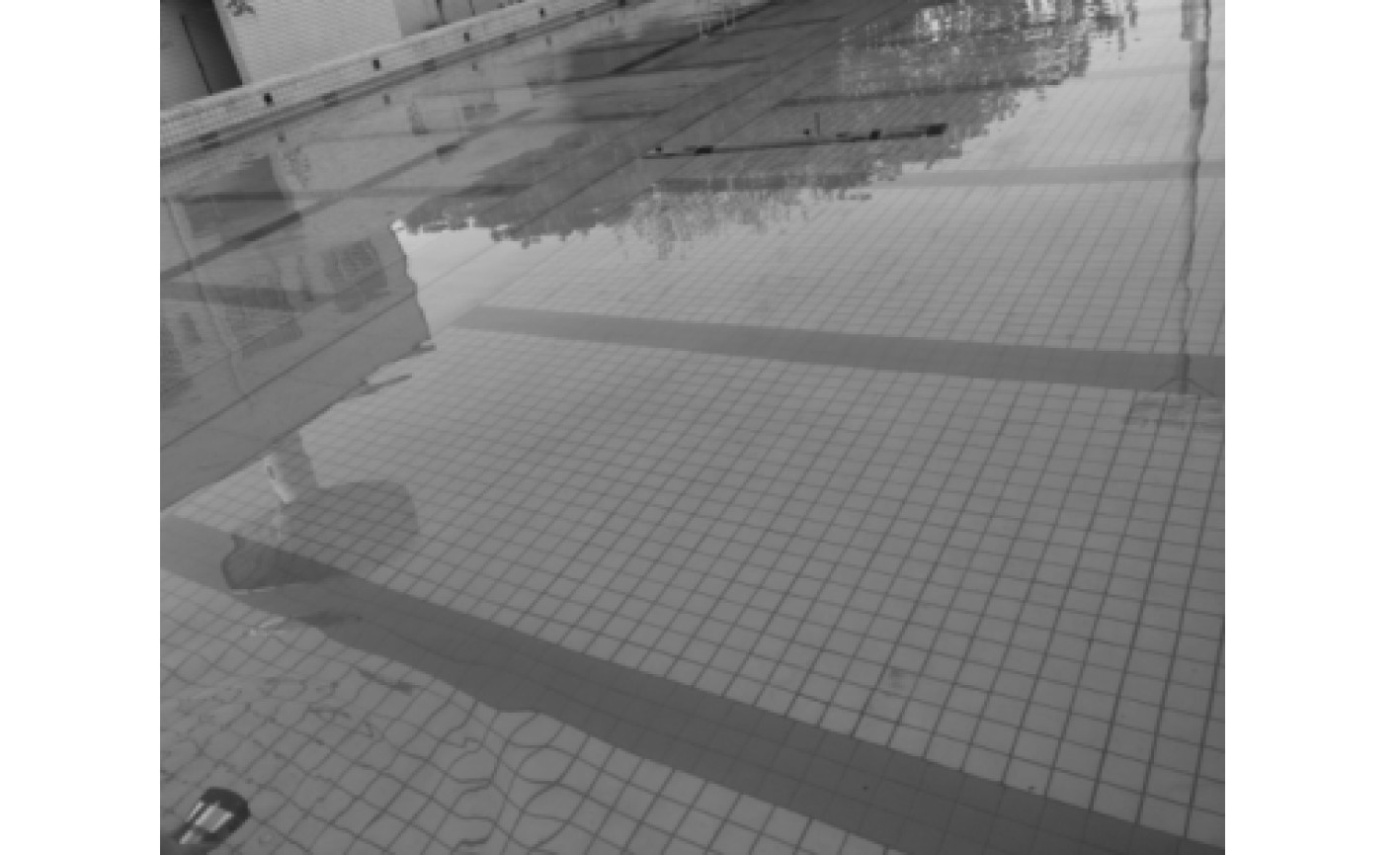图 1 目标物-AUV及实验场景 Fig. 1 Target-AUV and experimental scene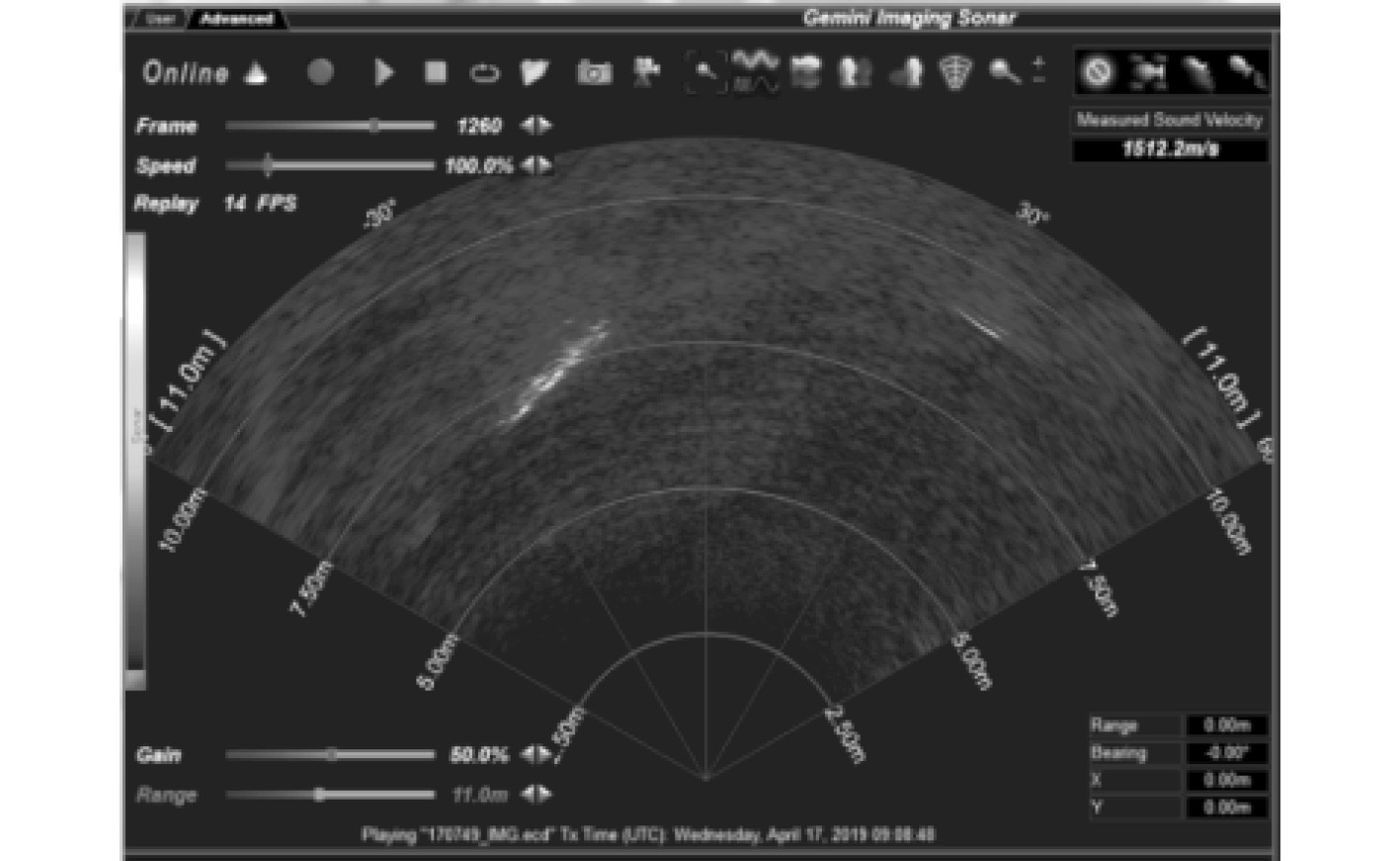图 2 原始声呐图像 Fig. 2 Original sonar image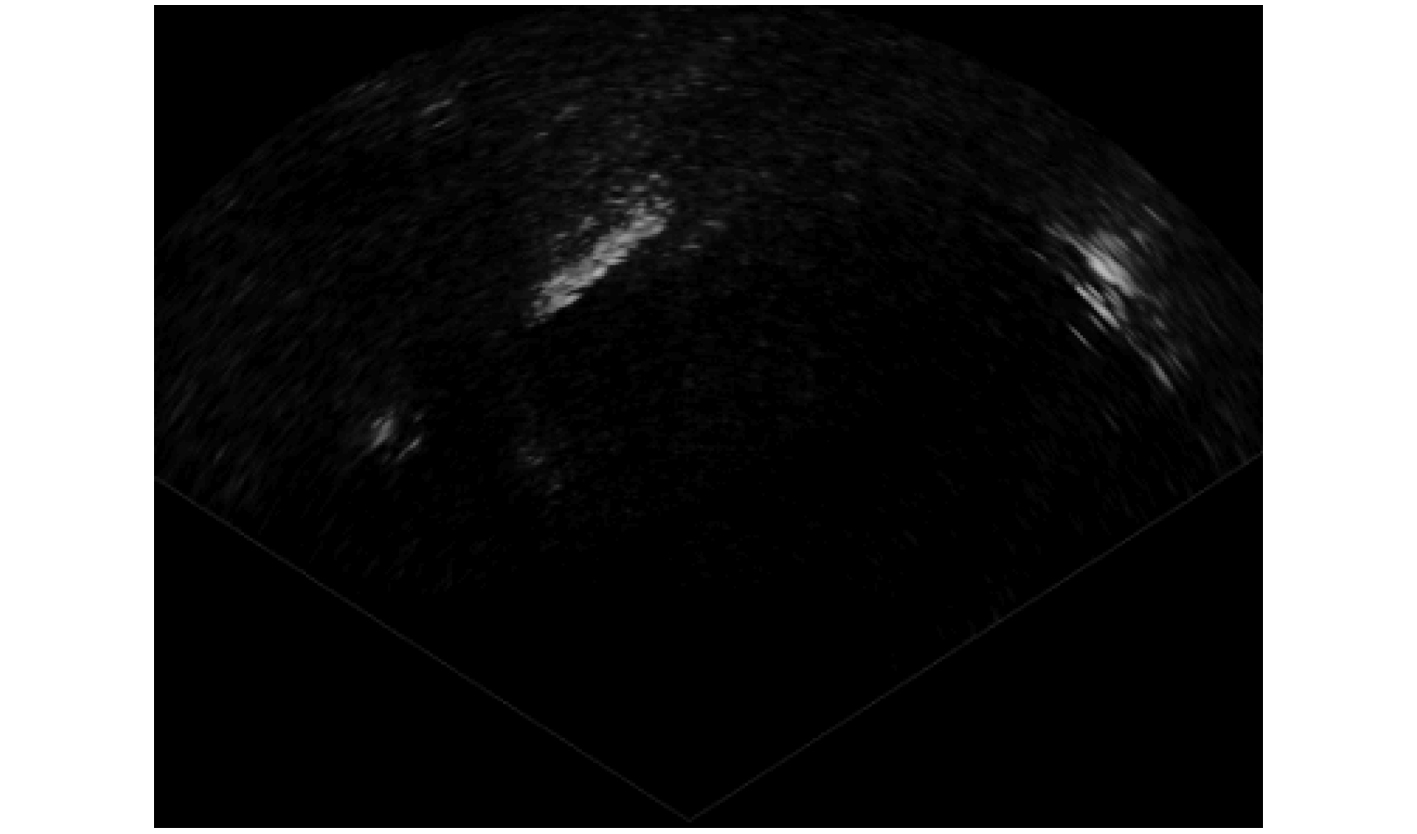图 3 灰度声呐图像 Fig. 3 Grayscale sonar image
1.1 标准中值滤波算法

1.2 改进的中值滤波算法

1）求像素点x4邻域内像素点灰度值的平均值，若其平均值为0，则直接将像素点x4的灰度值直接置为0；

2）将像素点{x0,x1,x2,x3,x4,x5,x6,x7,x8}分为3组，分别为{x0,x1,x2}，{x3,x4,x5}，{x6,x7,x8}；

3）对{x0,x1,x2}，{x3,x4,x5}，{x6,x7,x8}三组内的像素点进行降序排列，排序后的像素点表示为{y0,y1,y2}，{y3,y4,y5}，{y6,y7,y8}；

4）求出像素点y1y4y7的中值，即为所求邻域的中值。表 1 三种中值滤波所需要的的时间 Tab.1 Time required for three kinds of median filtering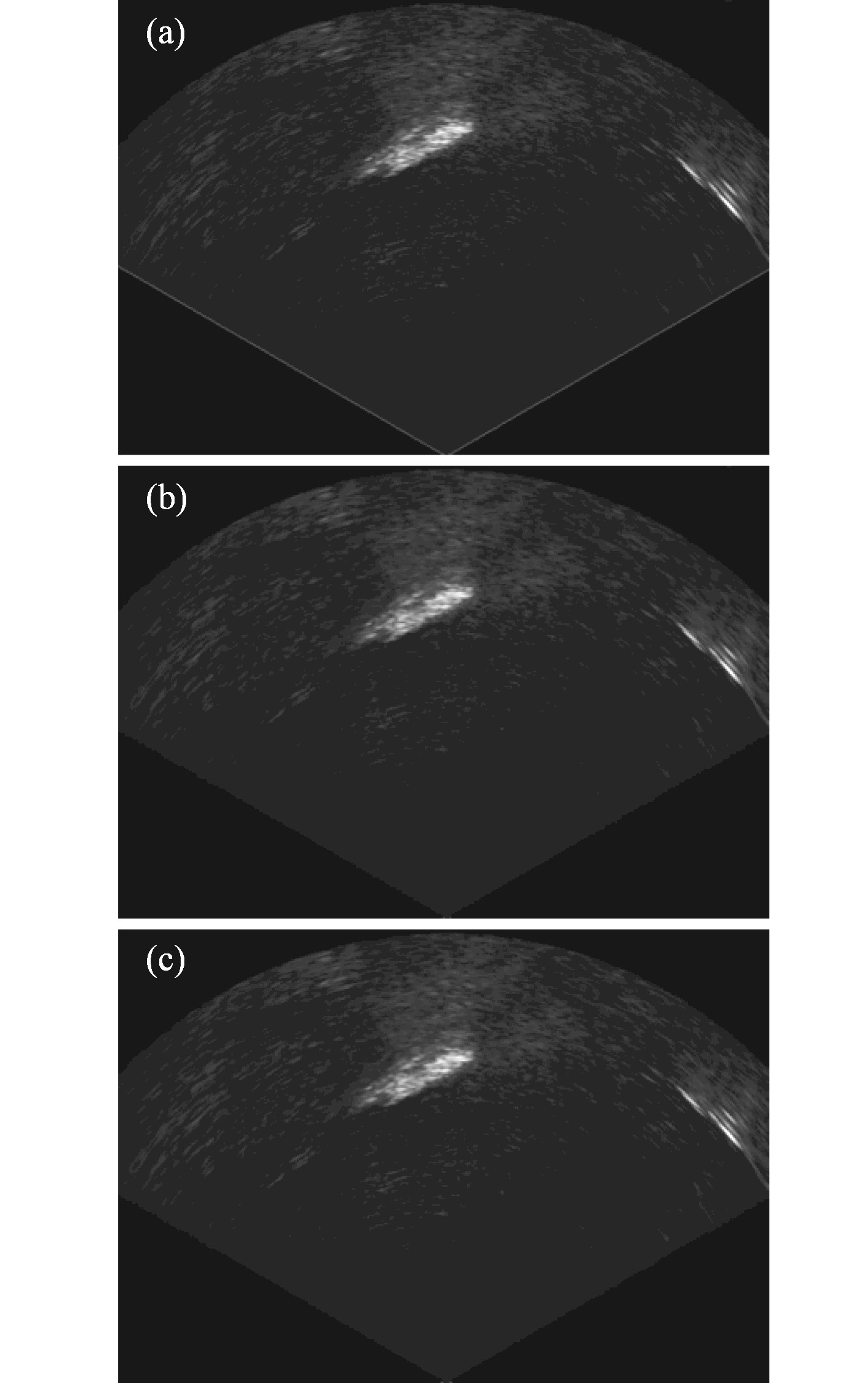图 4 原始声呐图像和3种中值滤波后的声呐图像 Fig. 4 The original sonar image and three median filtered sonar images
2 声呐图像增强

2.1 传统的模糊增强算法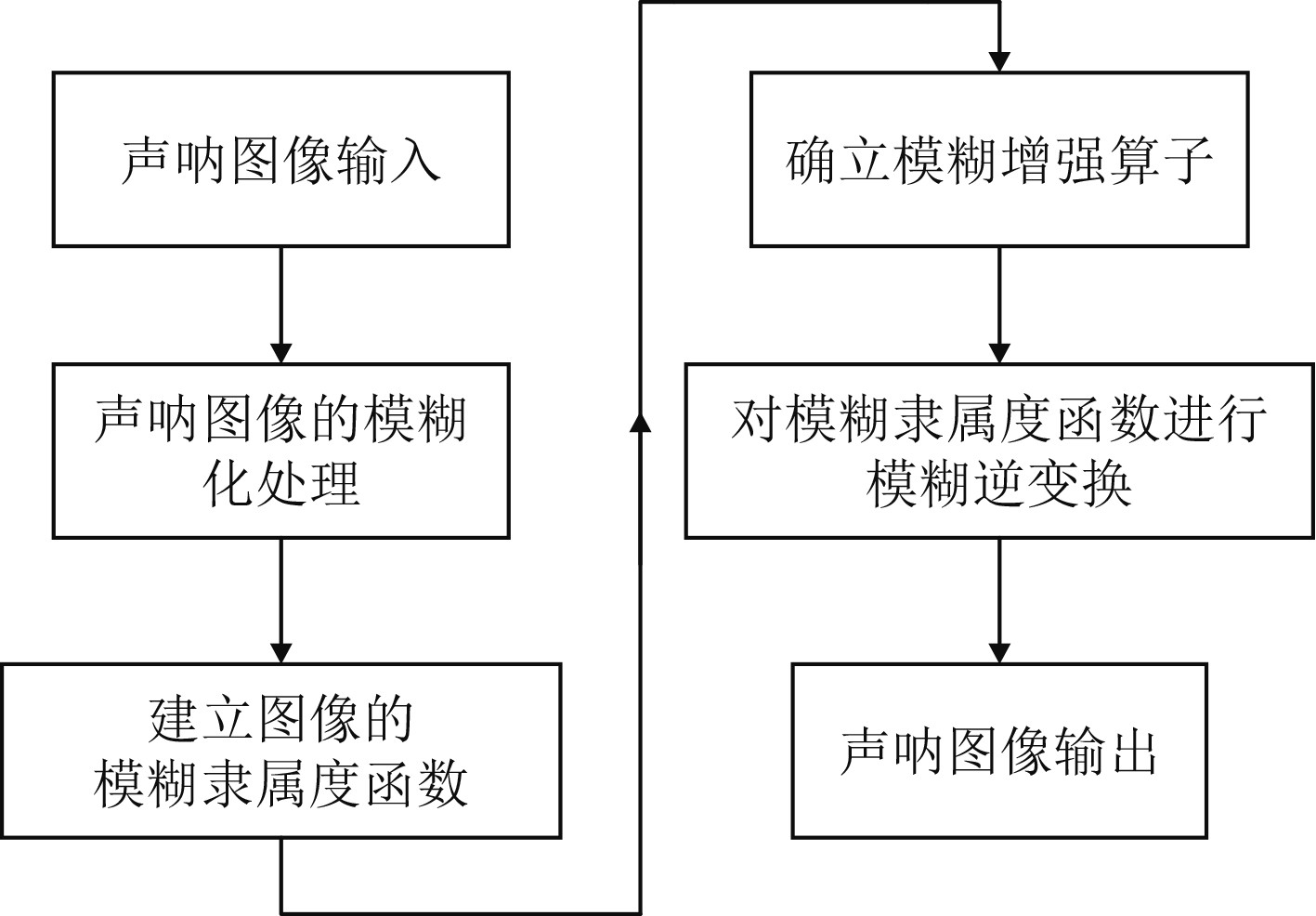图 5 传统模糊图像增强算法流程图 Fig. 5 Flow chart of traditional fuzzy image enhancement algorithm

1）将声呐图像从空间灰度域映射到声呐图像的模糊特征平面

 ${{X}} = \left| {\begin{array}{*{20}{c}} {\displaystyle\frac{{{u_{11}}}}{{{x_{11}}}}}&{\displaystyle\frac{{{u_{12}}}}{{{x_{12}}}}}& \ldots &{\displaystyle\frac{{{u_{1N}}}}{{{x_{1N}}}}} \\ {\displaystyle\frac{{{u_{21}}}}{{{x_{21}}}}}&{\displaystyle\frac{{{u_{22}}}}{{{x_{22}}}}}& \cdots &{\displaystyle\frac{{{u_{2N}}}}{{{x_{2N}}}}} \\ \vdots & \vdots & \vdots & \vdots \\ {\displaystyle\frac{{{u_{M1}}}}{{{x_{M1}}}}}&{\displaystyle\frac{{{u_{M2}}}}{{{x_{M2}}}}}& \cdots &{\displaystyle\frac{{{u_{MN}}}}{{{x_{MN}}}}} \end{array}} \right| = \bigcup\limits_{i = 1}^M {\bigcup\limits_{j = 1}^N {\frac{{{u_{ij}}}}{{{x_{ij}}}}} }{\text{。}}$ (1)

 ${\mu _{ij}} = G(x_{ij}^{}) = {[1 + \frac{{{x_{\max }} - {x_{ij}}}}{{Fd}}]^{ - {F_e}}}{\text{。}}$ (2)

2）声呐图像的模糊增强

 $u_{ij}' = {T^{(m)}}({u_{ij}}) = T\left( {{T^{(m - 1)}}({u_{ij}})} \right){\text{。}}$ (3)

 $T({u_{ij}}) = \left\{ {\begin{array}{*{20}{c}} {2{{({u_{ij}})}^2}} {\text{，}}\\ {1 - 2{{(1 - {u_{ij}})}^2}} {\text{。}} \end{array}} \right.$ (4)

3）声呐图像的模糊逆变换

 $x_{ij}' = {G^{ - 1}}(\mu _{_{ij}}') = {x_{\max }} - {F_d}[{(\mu _{_{ij}}')^{ - \frac{1}{{{F_e}}}}} - 1]{\text{。}}$ (5)

2.2 一种基于ln函数的模糊图像增强算法

 ${u_{ij}} = G({x_{ij}}) = \ln (1 + \frac{{(e - 1)({x_{ij}} - {x_{\min }})}}{{({x_{\max }} - {x_{\min }})}}){\text{。}}$ (6)

1）输入原始的声呐图像，计算声呐图像中像素点灰度值的最大值 ${x_{\max }}$ 和最小值 ${x_{\min }}$

2）通过式（6）将声呐图像从空间灰度域映射到模糊特征平面；

3）在将一幅声呐图像映射到模糊集合之后，需要利用模糊增强算子对声呐图像进行模糊增强处理。模糊增强算子下式：

 $u_{ij}' = {T^{(m)}}({u_{ij}}) = T\left( {{T^{(m - 1)}}({u_{ij}})} \right){\text{。}}$ (7)

 $T({u_{ij}}) = \left\{ {\begin{array}{*{20}{c}} {2{{({u_{ij}})}^2}}{\text{，}} \\ {1 - 2{{(1 - {u_{ij}})}^2}} {\text{。}} \end{array}} \right.$ (8)

3）对经模糊增算子增强后的模糊隶属度函数进行模糊逆变换，得到最终模糊增强后的声呐图像。

 $x_{ij}' = G_{ij}^{ - 1}(u_{ij}') = {{({e^{u_{ij}'}})({x_{\max }} - {x_{\min }})}/{(e - 1)}} + {x_{\min }}{\text{。}}$ (9)
2.3 增强效果对比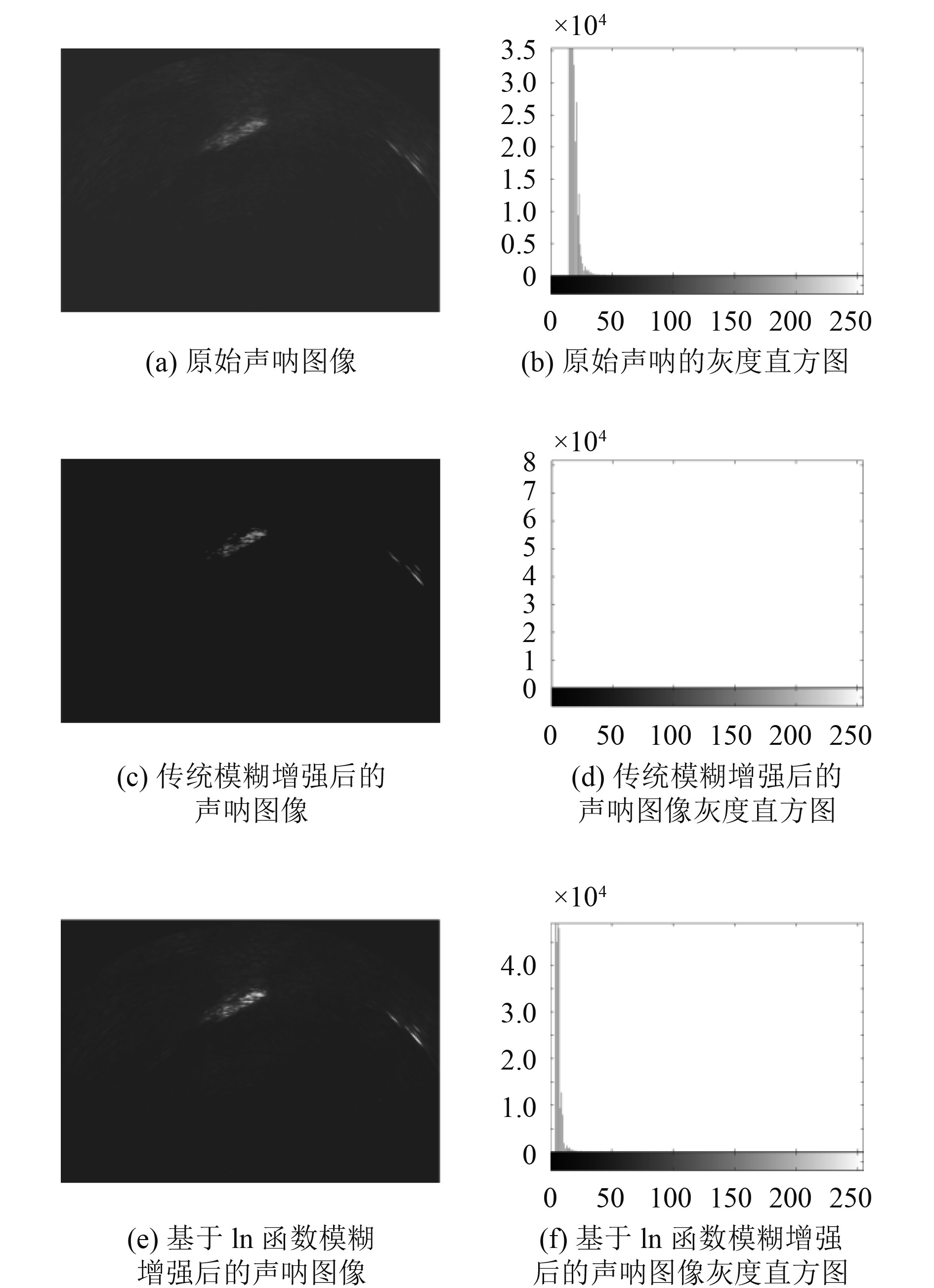图 6 声呐图像及其所对应的直方图 Fig. 6 Sonar image and its corresponding histogram
3 结　语

  凌宏杰, 曾庆军, 姚震球. 有缆遥控水下机器人的研究与发展趋势[J]. 中外船舶科技, 2018, 112(01): 5-12.  佚名. 目标物体的识别介质及识别方法:, CN 1791816 A[P]. 2006.  成像声纳目标检测与跟踪技术研究[D]. 哈尔滨: 哈尔滨工程大学, 2015.  马珊. 水下机器人前视声呐多目标跟踪技术研究[D]. 哈尔滨: 哈尔滨工程大学, 2016.  辛光红, 杨波. 基于声呐图像处理的船用水下目标识别技术研究[J]. 舰船科学技术, 2018(2x): 115-117.  Wen Long Jiang, Guang Lin Li, Wei Bing Luo. Application of improved median filtering algorithm to image de-noising[J]. Advanced Materials Research, 2014, 998: 838-841.  LI W, NI R, LI X, et al. Robust median filtering detection based on the difference of frequency residuals[J]. Multimedia Tools and Applications, 2018, 78(4): 8363-8381.  黄允浒, 吐尔洪江·阿布都克力木, 唐泉, 等. 一种基于à trous算法的遥感图像模糊集增强算法[J]. 计算机应用与软件, 2018, 35(3): 187-192.  基于模糊理论的医学图像增强算法研究[D]. 太原: 中北大学, 2017.  张家敏, 曾庆军, 朱志宇, 等. 自主式水下机器人控制系统及声呐目标识别[J]. 中国舰船研究, 2018, 13(06): 96-102.  王武兵, 张春燕. 基于计算机网络的船舶视频图像模糊细节增强算法[J]. 舰船科学技术, 2018, 40(18): 167-169.